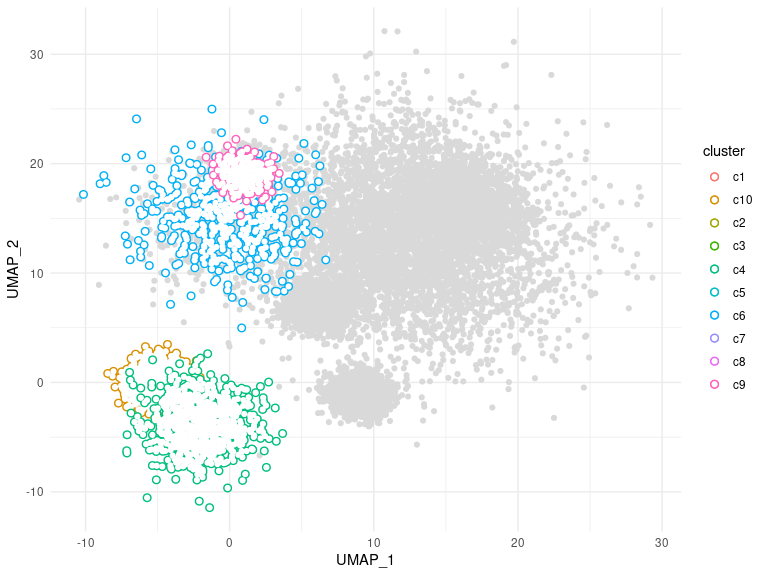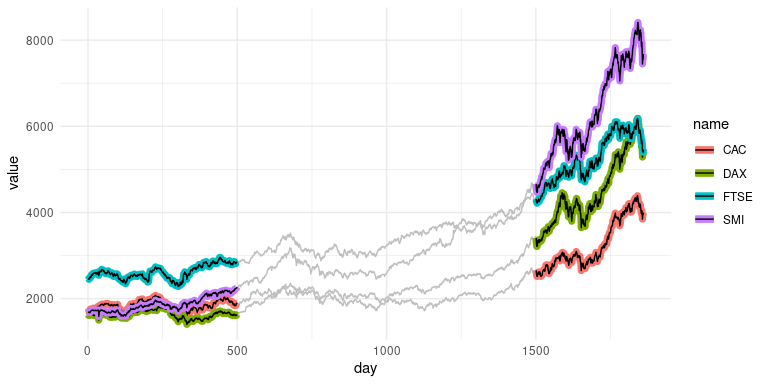# ggtrace

ggtrace provides ggplot2 geoms that allow groups of data points to be outlined or highlighted for emphasis. This is particularly useful when working with dense datasets that are prone to overplotting.

## Installation

You can install the released version from CRAN:

``install.packages("ggtrace")``

and the development version from GitHub:

``devtools::install_github("rnabioco/ggtrace")``

## Basic Usage

`geom_point_trace()` accepts graphical parameters normally passed to `ggplot2::geom_point()` to control the appearance of data points and outlines. The `trace_position` argument can be used to select specific sets of points to highlight. For more examples see the vignette.

``````library(ggplot2)
library(ggtrace)

ggplot(clusters, aes(UMAP_1, UMAP_2, color = cluster)) +
geom_point_trace(
trace_position    = signal < 0,
fill              = "white",
background_params = list(color = NA, fill = "grey85")
) +
theme_minimal()```````geom_line_trace()` accepts parameters normally passed to `ggplot2::geom_line()` with the following exceptions: `fill` controls the inner line color, `color` controls the outline color, and `stroke` controls outline width. Like `geom_point_trace()`, the `trace_position` argument can be used to select specific data points to highlight. For more examples see the vignette.

``````ggplot(stocks, aes(day, value, color = name)) +
geom_line_trace(
trace_position    = day < 500 | day > 1500,
stroke            = 1,
background_params = list(color = NA, fill = "grey75")
) +
theme_minimal()``````